# Sharp error bounds in approximating the Riemann-Stieltjes integral by a generalised trapezoid formula and applications

## Abstract

Sharp error bounds in approximating the Riemann-Stieltjes integral ${\int }_{a}^{b}f\left(t\right)\phantom{\rule{0.2em}{0ex}}du\left(t\right)$ with the generalised trapezoid formula $f\left(b\right)\left[u\left(b\right)-\frac{1}{b-a}{\int }_{a}^{b}u\left(s\right)\phantom{\rule{0.2em}{0ex}}ds\right]+f\left(a\right)\left[\frac{1}{b-a}{\int }_{a}^{b}u\left(s\right)\phantom{\rule{0.2em}{0ex}}ds-u\left(a\right)\right]$ are given for various pairs $\left(f,u\right)$ of functions. Applications for weighted integrals are also provided.

MSC: 26D15, 26D10, 41A55.

## 1 Introduction

In , in order to approximate the Riemann-Stieltjes integral ${\int }_{a}^{b}f\left(t\right)\phantom{\rule{0.2em}{0ex}}du\left(t\right)$ by the generalised trapezoid formula

$\left[u\left(b\right)-u\left(x\right)\right]f\left(b\right)+\left[u\left(x\right)-u\left(a\right)\right]f\left(a\right),\phantom{\rule{1em}{0ex}}x\in \left[a,b\right],$
(1.1)

the authors considered the error functional

$T\left(f,u;a,b;x\right):={\int }_{a}^{b}f\left(t\right)\phantom{\rule{0.2em}{0ex}}du\left(t\right)-\left[u\left(b\right)-u\left(x\right)\right]f\left(b\right)-\left[u\left(x\right)-u\left(a\right)\right]f\left(a\right)$
(1.2)

and proved that

$|T\left(f,u;a,b;x\right)|\le H{\left[\frac{1}{2}\left(b-a\right)+|x-\frac{a+b}{2}|\right]}^{r}\underset{a}{\overset{b}{\bigvee }}\left(f\right),\phantom{\rule{1em}{0ex}}x\in \left[a,b\right],$
(1.3)

provided that $f:\left[a,b\right]\to \mathbb{R}$ is of bounded variation on $\left[a,b\right]$ and u is of r-H-Hölder type, that is, $u:\left[a,b\right]\to \mathbb{R}$ satisfies the condition $|u\left(t\right)-u\left(s\right)|\le H|t-s{|}^{r}$ for any $t,s\in \left[a,b\right]$, where $r\in \left(0,1\right]$ and $H>0$ are given.

The dual case, namely, when f is of q-K-Hölder type and u is of bounded variation, has been considered by the authors in  in which they obtained the bound: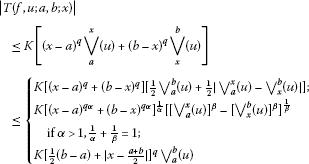(1.4)

for any $x\in \left[a,b\right]$.

The case where f is monotonic and u is of r-H-Hölder type, which provides a refinement for (1.3), and respectively the case where u is monotonic and f of q-K-Hölder type were considered by Cheung and Dragomir in , while the case where one function was of Hölder type and the other was Lipschitzian was considered in . For other recent results in estimating the error $T\left(f,u;a,b,x\right)$ for absolutely continuous integrands f and integrators u of bounded variation, see  and .

The main aim of the present paper is to investigate the error bounds in approximating the Stieltjes integral by a different generalised trapezoid rule than the one from (1.1) in which the value $u\left(x\right)$, $x\in \left[a,b\right]$ is replaced with the integral mean $\frac{1}{b-a}{\int }_{a}^{b}u\left(s\right)\phantom{\rule{0.2em}{0ex}}ds$. Applications in approximating the weighted integrals ${\int }_{a}^{b}h\left(t\right)f\left(t\right)\phantom{\rule{0.2em}{0ex}}dt$ are also provided.

## 2 Representation results

We consider the following error functional ${T}_{g}\left(f;u\right)$ in approximating the Riemann-Stieltjes integral ${\int }_{a}^{b}f\left(t\right)\phantom{\rule{0.2em}{0ex}}du\left(t\right)$ by the generalised trapezoid formula: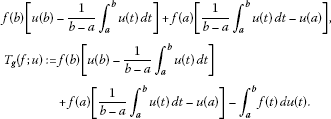(2.1)

If we consider the associated functions ${\mathrm{\Phi }}_{f}$, ${\mathrm{\Gamma }}_{f}$ and ${\mathrm{\Delta }}_{f}$ defined by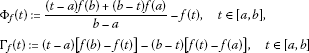and

${\mathrm{\Delta }}_{f}\left(t\right):=\frac{f\left(b\right)-f\left(t\right)}{b-t}-\frac{f\left(t\right)-f\left(a\right)}{t-a},\phantom{\rule{1em}{0ex}}t\in \left(a,b\right),$

then we observe that

(2.2)

The following representation result can be stated.

Theorem 1 Let $f,u:\left[a,b\right]\to \mathbb{R}$ be bounded on $\left[a,b\right]$ and such that the Riemann-Stieltjes integral ${\int }_{a}^{b}f\left(t\right)\phantom{\rule{0.2em}{0ex}}du\left(t\right)$ and the Riemann integral ${\int }_{a}^{b}u\left(t\right)\phantom{\rule{0.2em}{0ex}}dt$ exist. Then we have the identities

$\begin{array}{rcl}{T}_{g}\left(f;u\right)& =& {\int }_{a}^{b}{\mathrm{\Phi }}_{f}\left(t\right)\phantom{\rule{0.2em}{0ex}}du\left(t\right)\\ =& \frac{1}{b-a}{\int }_{a}^{b}{\mathrm{\Gamma }}_{f}\left(t\right)\phantom{\rule{0.2em}{0ex}}du\left(t\right)\\ =& \frac{1}{b-a}{\int }_{a}^{b}\left(b-t\right)\left(t-a\right){\mathrm{\Delta }}_{f}\left(t\right)\phantom{\rule{0.2em}{0ex}}du\left(t\right)\\ =& D\left(u;f\right),\end{array}$
(2.3)

where

$D\left(u;f\right)={\int }_{a}^{b}u\left(t\right)\phantom{\rule{0.2em}{0ex}}df\left(t\right)-\left[f\left(b\right)-f\left(a\right)\right]\cdot \frac{1}{b-a}{\int }_{a}^{b}u\left(t\right)\phantom{\rule{0.2em}{0ex}}dt.$
(2.4)

Proof

Integrating the Riemann-Stieltjes integral by parts, we have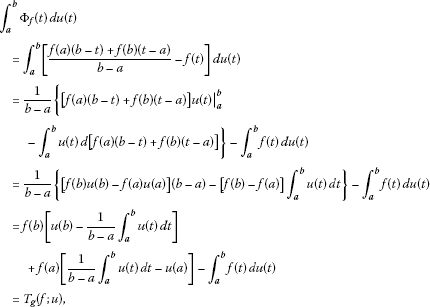and the first equality in (2.3) is proved.

The second and third identity is obvious by the relation (2.2).

For the last equality, we use the fact that for any $g,h:\left[a,b\right]\to \mathbb{R}$ bounded functions for which the Riemann-Stieltjes integral ${\int }_{a}^{b}h\left(t\right)\phantom{\rule{0.2em}{0ex}}dg\left(t\right)$ and the Riemann integral ${\int }_{a}^{b}g\left(t\right)\phantom{\rule{0.2em}{0ex}}dt$ exist, we have the representation (see, for instance, )

$D\left(g;h\right)={\int }_{a}^{b}{\mathrm{\Phi }}_{h}\left(t\right)\phantom{\rule{0.2em}{0ex}}dg\left(t\right).$
(2.5)

The proof is now complete. □

In the case where u is an integral, the following identity can be stated.

Corollary 1 Let $p,h:\left[a,b\right]\to \mathbb{R}$ be continuous on $\left[a,b\right]$ and $f:\left[a,b\right]\to \mathbb{R}$ be Riemann integrable. Then we have the identity

$\begin{array}{rcl}{T}_{g}\left(f;{\int }_{a}ph\right)& =& \frac{1}{b-a}\left[f\left(b\right)\cdot {\int }_{a}^{b}\left(t-a\right)p\left(t\right)h\left(t\right)\phantom{\rule{0.2em}{0ex}}dt+f\left(a\right)\cdot {\int }_{a}^{b}\left(b-t\right)p\left(t\right)h\left(t\right)\phantom{\rule{0.2em}{0ex}}dt\right]\\ -{\int }_{a}^{b}p\left(t\right)f\left(t\right)h\left(t\right)\phantom{\rule{0.2em}{0ex}}dt\\ =& {\int }_{a}^{b}{\mathrm{\Phi }}_{f}\left(t\right)p\left(t\right)h\left(t\right)\phantom{\rule{0.2em}{0ex}}dt.\end{array}$
(2.6)

Proof Since p and h are continuous, the function $u\left(t\right)={\int }_{a}^{t}p\left(s\right)h\left(s\right)\phantom{\rule{0.2em}{0ex}}ds$ is differentiable and ${u}^{\mathrm{\prime }}\left(t\right)=p\left(t\right)h\left(t\right)$ for each $t\in \left(a,b\right)$.

Integrating by parts, we have

$\begin{array}{rcl}{\int }_{a}^{b}u\left(t\right)\phantom{\rule{0.2em}{0ex}}dt& =& \left({\int }_{a}^{t}p\left(s\right)h\left(s\right)\phantom{\rule{0.2em}{0ex}}ds\right)\cdot t{|}_{a}^{b}-{\int }_{a}^{b}tp\left(t\right)h\left(t\right)\phantom{\rule{0.2em}{0ex}}dt\\ =& b{\int }_{a}^{b}p\left(s\right)h\left(s\right)\phantom{\rule{0.2em}{0ex}}ds-{\int }_{a}^{b}tp\left(t\right)h\left(t\right)\phantom{\rule{0.2em}{0ex}}dt\\ =& {\int }_{a}^{b}\left(b-t\right)p\left(t\right)h\left(t\right)\phantom{\rule{0.2em}{0ex}}dt.\end{array}$

Since

$\begin{array}{rcl}u\left(b\right)-\frac{1}{b-a}{\int }_{a}^{b}u\left(t\right)\phantom{\rule{0.2em}{0ex}}dt& =& {\int }_{a}^{b}p\left(t\right)h\left(t\right)\phantom{\rule{0.2em}{0ex}}dt-\frac{1}{b-a}{\int }_{a}^{b}\left(b-t\right)p\left(t\right)h\left(t\right)\phantom{\rule{0.2em}{0ex}}dt\\ =& \frac{1}{b-a}{\int }_{a}^{b}\left(t-a\right)p\left(t\right)h\left(t\right)\phantom{\rule{0.2em}{0ex}}dt,\end{array}$

then, by the definition of ${T}_{g}$ in (2.1), we deduce the first part of (2.6).

The second part of (2.6) follows by (2.3). □

Remark 1 In the particular case $p\left(t\right)=1$, $t\in \left[a,b\right]$, we have the equality

$\begin{array}{rcl}{T}_{g}\left(f;{\int }_{a}h\right)& =& \frac{1}{b-a}\left[f\left(b\right)\cdot {\int }_{a}^{b}\left(t-a\right)h\left(t\right)\phantom{\rule{0.2em}{0ex}}dt+f\left(a\right)\cdot {\int }_{a}^{b}\left(b-t\right)h\left(t\right)\phantom{\rule{0.2em}{0ex}}dt\right]-{\int }_{a}^{b}f\left(t\right)h\left(t\right)\phantom{\rule{0.2em}{0ex}}dt\\ =& {\int }_{a}^{b}{\mathrm{\Phi }}_{f}\left(t\right)h\left(t\right)\phantom{\rule{0.2em}{0ex}}dt=\frac{1}{b-a}{\int }_{a}^{b}{\mathrm{\Gamma }}_{f}\left(t\right)h\left(t\right)\phantom{\rule{0.2em}{0ex}}dt.\end{array}$
(2.7)

## 3 Some inequalities for f-convex

The following result concerning the nonnegativity of the error functional ${T}_{g}\left(\cdot ;\cdot \right)$ can be stated.

Theorem 2 If u is monotonic nonincreasing and $f:\left[a,b\right]\to \mathbb{R}$ is such that the Riemann-Stieltjes integral ${\int }_{a}^{b}f\left(t\right)\phantom{\rule{0.2em}{0ex}}du\left(t\right)$ exists and

$\frac{f\left(b\right)-f\left(t\right)}{b-t}\ge \frac{f\left(t\right)-f\left(a\right)}{t-a}\phantom{\rule{1em}{0ex}}\mathit{\text{for any}}\phantom{\rule{0.1em}{0ex}}t\in \left(a,b\right),$
(3.1)

then ${T}_{g}\left(f;u\right)\ge 0$ or, equivalently,

$f\left(b\right)\left[u\left(b\right)-\frac{1}{b-a}{\int }_{a}^{b}u\left(t\right)\phantom{\rule{0.2em}{0ex}}dt\right]+f\left(a\right)\left[\frac{1}{b-a}{\int }_{a}^{b}u\left(t\right)\phantom{\rule{0.2em}{0ex}}dt-u\left(a\right)\right]\ge {\int }_{a}^{b}f\left(t\right)\phantom{\rule{0.2em}{0ex}}du\left(t\right).$
(3.2)

A sufficient condition for (3.1) to hold is that f is convex on $\left[a,b\right]$.

Proof The condition (3.1) is equivalent with the fact that ${\mathrm{\Delta }}_{f}\left(t\right)\ge 0$ for any $t\in \left(a,b\right)$ and then, by the equality

${T}_{g}\left(f;u\right)=\frac{1}{b-a}{\int }_{a}^{b}\left(b-t\right)\left(t-a\right){\mathrm{\Delta }}_{f}\left(t\right)\phantom{\rule{0.2em}{0ex}}du\left(t\right),$

we deduce that ${T}_{g}\left(f;u\right)\ge 0$.

If f is convex, then

$\frac{t-a}{b-a}f\left(b\right)+\frac{b-t}{b-a}f\left(a\right)\ge f\left[\left(\frac{t-a}{b-a}\right)b+\left(\frac{b-t}{b-a}\right)a\right]=f\left(t\right),$

which shows that ${\mathrm{\Phi }}_{f}\left(t\right)\ge 0$, namely, the condition (3.1) is satisfied. □

Corollary 2 Let $p,h:\left[a,b\right]\to \mathbb{R}$ be continuous on $\left[a,b\right]$ and $f:\left[a,b\right]\to \mathbb{R}$ be Riemann integrable. If $p\left(t\right)h\left(t\right)\ge 0$ for any $t\in \left[a,b\right]$ and f satisfies (3.1) or, sufficiently, f is convex on $\left[a,b\right]$, then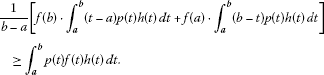(3.3)

We are now able to provide some new results.

Theorem 3 Assume that p and h are continuous and synchronous (asynchronous) on $\left(a,b\right)$, i.e.,

$\left(p\left(t\right)-p\left(s\right)\right)\left(h\left(t\right)-h\left(s\right)\right)\phantom{\rule{0.25em}{0ex}}\ge \left(\le \right)\phantom{\rule{0.25em}{0ex}}0\phantom{\rule{1em}{0ex}}\mathit{\text{for any}}\phantom{\rule{0.1em}{0ex}}t,s\in \left[a,b\right].$
(3.4)

If f satisfies (3.1) and is Riemann integrable on $\left[a,b\right]$ (or sufficiently, f is convex on $\left[a,b\right]$), then

${T}_{g}\left(f;{\int }_{a}p\right)\cdot {T}_{g}\left(f;{\int }_{a}h\right)\phantom{\rule{0.25em}{0ex}}\le \left(\ge \right)\phantom{\rule{0.25em}{0ex}}{T}_{g}\left(f;{\int }_{a}1\right)\cdot {T}_{g}\left(f;{\int }_{a}ph\right),$
(3.5)

where

${T}_{g}\left(f;{\int }_{a}1\right)=\frac{f\left(a\right)+f\left(b\right)}{2}\left(b-a\right)-{\int }_{a}^{b}f\left(t\right)\phantom{\rule{0.2em}{0ex}}dt.$
(3.6)

Proof

We use the Čebyšev inequality

${\int }_{a}^{b}\alpha \left(t\right)\phantom{\rule{0.2em}{0ex}}dt{\int }_{a}^{b}\alpha \left(t\right)p\left(t\right)h\left(t\right)\phantom{\rule{0.2em}{0ex}}dt\phantom{\rule{0.25em}{0ex}}\ge \left(\le \right)\phantom{\rule{0.25em}{0ex}}{\int }_{a}^{b}\alpha \left(t\right)p\left(t\right)\phantom{\rule{0.2em}{0ex}}dt{\int }_{a}^{b}\alpha \left(t\right)h\left(t\right)\phantom{\rule{0.2em}{0ex}}dt,$
(3.7)

which holds for synchronous (asynchronous) functions p, h and nonnegative α for which the involved integrals exist.

Now, on applying the Čebyšev inequality (3.7) for $\alpha \left(t\right)={\mathrm{\Phi }}_{f}\left(t\right)\ge 0$ and utilising the representation result (2.6), we deduce the desired inequality (3.5). □

We also have the following theorem.

Theorem 4 Assume that $f:\left[a,b\right]\to \mathbb{R}$ is Riemann integrable and satisfies (3.1) (or sufficiently, f is concave on $\left[a,b\right]$). Then, for $p,h:\left[a,b\right]\to \mathbb{R}$ continuous, we have

$|{T}_{g}\left(f;{\int }_{a}ph\right)|\le \underset{t\in \left[a,b\right]}{sup}|h\left(t\right)|{T}_{g}\left(f;{\int }_{a}|p|\right)$
(3.8)

and

$|{T}_{g}\left(f;{\int }_{a}ph\right)|\le {\left[{T}_{g}\left(f;{\int }_{a}|p{|}^{\alpha }\right)\right]}^{\frac{1}{\alpha }}{\left[{T}_{g}\left(f;{\int }_{a}|h{|}^{\beta }\right)\right]}^{\frac{1}{\beta }},$
(3.9)

where $\alpha >1$, $\frac{1}{\alpha }+\frac{1}{\beta }=1$. In particular, we have

$|{T}_{g}\left(f;{\int }_{a}ph\right){|}^{2}\le {T}_{g}\left(f;{\int }_{a}|p{|}^{2}\right){T}_{g}\left(f;{\int }_{a}|h{|}^{2}\right).$
(3.10)

Proof

Observe that

$\begin{array}{rcl}|{T}_{g}\left(f;{\int }_{a}ph\right)|& =& |{\int }_{a}^{b}{\mathrm{\Phi }}_{f}\left(t\right)p\left(t\right)h\left(t\right)\phantom{\rule{0.2em}{0ex}}dt|\\ \le & {\int }_{a}^{b}|{\mathrm{\Phi }}_{f}\left(t\right)p\left(t\right)h\left(t\right)|\phantom{\rule{0.2em}{0ex}}dt\\ =& {\int }_{a}^{b}{\mathrm{\Phi }}_{f}\left(t\right)|p\left(t\right)||h\left(t\right)|\phantom{\rule{0.2em}{0ex}}dt\\ \le & \underset{t\in \left[a,b\right]}{sup}|h\left(t\right)|{\int }_{a}^{b}{\mathrm{\Phi }}_{f}\left(t\right)|p\left(t\right)|\phantom{\rule{0.2em}{0ex}}dt\\ =& \underset{t\in \left[a,b\right]}{sup}|h\left(t\right)|{T}_{g}\left(f;{\int }_{a}|p|\right)\end{array}$

and the inequality (3.8) is proved.

Further, by the Hölder inequality, we also have

$\begin{array}{rcl}|{T}_{g}\left(f;{\int }_{a}ph\right)|& \le & {\int }_{a}^{b}{\mathrm{\Phi }}_{f}\left(t\right)|p\left(t\right)||h\left(t\right)|\phantom{\rule{0.2em}{0ex}}dt\\ \le & {\left({\int }_{a}^{b}{\mathrm{\Phi }}_{f}\left(t\right)|p\left(t\right){|}^{\alpha }\phantom{\rule{0.2em}{0ex}}dt\right)}^{\frac{1}{\alpha }}{\left({\int }_{a}^{b}{\mathrm{\Phi }}_{f}\left(t\right)|h\left(t\right){|}^{\beta }\phantom{\rule{0.2em}{0ex}}dt\right)}^{\frac{1}{\beta }}\\ =& {\left[{T}_{g}\left(f;{\int }_{a}|p{|}^{\alpha }\right)\right]}^{\frac{1}{\alpha }}{\left[{T}_{g}\left(f;{\int }_{a}|h{|}^{\beta }\right)\right]}^{\frac{1}{\beta }}\end{array}$

for $\alpha >1$, $\frac{1}{\alpha }+\frac{1}{\beta }=1$, and the theorem is proved. □

Remark 2 The above result can be useful for providing some error estimates in approximating the weighted integral ${\int }_{a}^{b}h\left(t\right)f\left(t\right)\phantom{\rule{0.2em}{0ex}}dt$ by the generalised trapezoid rule

$\frac{1}{b-a}\left[f\left(b\right)\cdot {\int }_{a}^{b}\left(t-a\right)h\left(t\right)\phantom{\rule{0.2em}{0ex}}dt+f\left(a\right)\cdot {\int }_{a}^{b}\left(b-t\right)h\left(t\right)\phantom{\rule{0.2em}{0ex}}dt\right]$

as follows:(3.11)

provided f satisfies (3.1) and is Riemann integrable (or sufficiently, convex on $\left[a,b\right]$), which is continuous on $\left[a,b\right]$.

If $h\left(t\right)=|w\left(t\right){|}^{\frac{1}{\beta }}$, $t\in \left[a,b\right]$, then for some f, we also have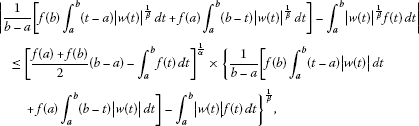(3.12)

with $\alpha >1$, $\frac{1}{\alpha }+\frac{1}{\beta }=1$.

Finally, we can state the following Jensen type inequality for the error functional ${T}_{g}\left(f;{\int }_{a}^{b}h\right)$.

Theorem 5 Assume $f:\left[a,b\right]\to \mathbb{R}$ is Riemann integrable and satisfies (3.1) (or sufficiently, f is convex on $\left[a,b\right]$), while $h:\left[a,b\right]\to \mathbb{R}$ is continuous. If $F:\mathbb{R}\to \mathbb{R}$ is convex (concave), then

$F\left(\frac{{T}_{g}\left(f;{\int }_{a}^{b}h\right)}{{T}_{g}\left(f;{\int }_{a}^{b}1\right)}\right)\phantom{\rule{0.25em}{0ex}}\le \left(\ge \right)\phantom{\rule{0.25em}{0ex}}\frac{{T}_{g}\left(f;{\int }_{a}^{b}F\circ h\right)}{{T}_{g}\left(f;{\int }_{a}^{b}1\right)}.$
(3.13)

Proof

By the use of Jensen’s integral inequality, we have

$F\left(\frac{{\int }_{a}^{b}{\mathrm{\Phi }}_{f}\left(t\right)h\left(t\right)\phantom{\rule{0.2em}{0ex}}dt}{{\int }_{a}^{b}{\mathrm{\Phi }}_{f}\left(t\right)\phantom{\rule{0.2em}{0ex}}dt}\right)\phantom{\rule{0.25em}{0ex}}\le \left(\ge \right)\phantom{\rule{0.25em}{0ex}}\frac{{\int }_{a}^{b}{\mathrm{\Phi }}_{f}\left(t\right)F\left(h\left(t\right)\right)\phantom{\rule{0.2em}{0ex}}dt}{{\int }_{a}^{b}{\mathrm{\Phi }}_{f}\left(t\right)\phantom{\rule{0.2em}{0ex}}dt}.$
(3.14)

Since, by the identity (2.6), we have

${\int }_{a}^{b}{\mathrm{\Phi }}_{f}\left(t\right)F\left(h\left(t\right)\right)\phantom{\rule{0.2em}{0ex}}dt={T}_{g}\left(f;{\int }_{a}^{b}F\circ h\right),$

then (3.14) is equivalent with the desired result (3.13). □

## 4 Sharp bounds via Grüss type inequalities

Due to the identity (2.3), in which the error bound ${T}_{g}\left(f;u\right)$ can be represented as $D\left(u;f\right)$, where

$D\left(u;f\right)={\int }_{a}^{b}u\left(t\right)\phantom{\rule{0.2em}{0ex}}df\left(t\right)-\left[f\left(b\right)-f\left(a\right)\right]\cdot \frac{1}{b-a}{\int }_{a}^{b}u\left(t\right)\phantom{\rule{0.2em}{0ex}}dt,$

is a Grüss type functional introduced in , any sharp bound for $D\left(u;f\right)$ will be a sharp bound for ${T}_{g}\left(f;u\right)$.

We can state the following result.

Theorem 6 Let $f,u:\left[a,b\right]\to \mathbb{R}$ be bounded functions on $\left[a,b\right]$.

(i) If there exist constants n, N such that $n\le u\left(t\right)\le N$ for any $t\in \left[a,b\right]$, u is Riemann integrable and f is K-Lipschitzian ($K>0$), then

$|{T}_{g}\left(f;u\right)|\le \frac{1}{2}K\left(N-n\right)\left(b-a\right).$
(4.1)

The constant $\frac{1}{2}$ is best possible in (4.1).

(ii) If f is of bounded variation and u is S-Lipschitzian ($S>0$), then

$|{T}_{g}\left(f;u\right)|\le \frac{1}{2}S\left(b-a\right)\underset{a}{\overset{b}{\bigvee }}\left(f\right).$
(4.2)

The constant $\frac{1}{2}$ is best possible in (4.2)

(iii) If f is monotonic nondecreasing and u is S-Lipschitzian, then

$\begin{array}{rcl}|{T}_{g}\left(f;u\right)|& \le & \frac{1}{2}S\left(b-a\right)\left[f\left(b\right)-f\left(a\right)-P\left(f\right)\right]\\ \le & \frac{1}{2}S\left(b-a\right)\left[f\left(b\right)-f\left(a\right)\right],\end{array}$
(4.3)

where

$P\left(f\right)=\frac{4}{{\left(b-a\right)}^{2}}{\int }_{a}^{b}\left(t-\frac{a+b}{2}\right)f\left(t\right)\phantom{\rule{0.2em}{0ex}}dt.$

The constant $\frac{1}{2}$ is best possible in both inequalities.

(iv) If f is monotonic nondecreasing and u is of bounded variation and such that the Riemann-Stieltjes integral ${\int }_{a}^{b}f\left(t\right)\phantom{\rule{0.2em}{0ex}}du\left(t\right)$ exists, then

$|{T}_{g}\left(f;u\right)|\le \left[f\left(b\right)-f\left(a\right)-Q\left(f\right)\right]\underset{a}{\overset{b}{\bigvee }}\left(u\right),$
(4.4)

where

$Q\left(f\right):=\frac{1}{b-a}{\int }_{a}^{b}sgn\left(t-\frac{a+b}{2}\right)f\left(t\right)\phantom{\rule{0.2em}{0ex}}dt.$

The inequality (4.4) is sharp.

(v) If f is continuous and convex on $\left[a,b\right]$ and u is of bounded variation on $\left[a,b\right]$, then

$|{T}_{g}\left(f;u\right)|\le \frac{1}{4}\left[{f}_{-}^{\mathrm{\prime }}\left(b\right)-{f}_{+}^{\mathrm{\prime }}\left(a\right)\right]\underset{a}{\overset{b}{\bigvee }}\left(u\right).$
(4.5)

The constant $\frac{1}{4}$ is sharp (if ${f}_{-}^{\mathrm{\prime }}\left(b\right)$ and ${f}_{+}^{\mathrm{\prime }}\left(a\right)$ are finite).

(vi) If $f:\left[a,b\right]\to \mathbb{R}$ is continuous and convex on $\left[a,b\right]$ and u is monotonic nondecreasing on $\left[a,b\right]$, then

(4.6)

The constants 2 and $\frac{1}{2}$ are best possible in (4.6) (if ${f}_{-}^{\mathrm{\prime }}\left(b\right)$ and ${f}_{+}^{\mathrm{\prime }}\left(a\right)$ are finite).

Proof The inequality (4.1) follows from the inequality (2.5) in  applied to $D\left(u;f\right)$, while (4.2) comes from (1.3) of . The inequalities (4.3) and (4.4) follow from , while (4.5) and (4.6) are valid via the inequalities (2.8) and (2.1) from  applied to the functional $D\left(u;f\right)$. The details are omitted. □

If we consider the error functional in approximating the weighted integral ${\int }_{a}^{b}h\left(t\right)f\left(t\right)\phantom{\rule{0.2em}{0ex}}dt$ by the generalised trapezoid formula,

$\frac{1}{b-a}\left[f\left(b\right)\cdot {\int }_{a}^{b}\left(t-a\right)h\left(t\right)\phantom{\rule{0.2em}{0ex}}dt+f\left(a\right)\cdot {\int }_{a}^{b}\left(b-t\right)h\left(t\right)\phantom{\rule{0.2em}{0ex}}dt\right],$

$\begin{array}{rcl}E\left(f;h\right)& :=& {T}_{g}\left(f;{\int }_{a}^{b}h\right)\\ =& \frac{1}{b-a}\left[f\left(b\right)\cdot {\int }_{a}^{b}\left(t-a\right)h\left(t\right)\phantom{\rule{0.2em}{0ex}}dt+f\left(a\right)\cdot {\int }_{a}^{b}\left(b-t\right)h\left(t\right)\phantom{\rule{0.2em}{0ex}}dt\right]\\ -{\int }_{a}^{b}h\left(t\right)f\left(t\right)\phantom{\rule{0.2em}{0ex}}dt,\end{array}$
(4.7)

then the following corollary provides various sharp bounds for the absolute value of $E\left(f;h\right)$.

Corollary 3 Assume that f and u are Riemann integrable on $\left[a,b\right]$.

(i) If there exist constants γ, Γ such that $\gamma \le {\int }_{a}^{t}h\left(s\right)\phantom{\rule{0.2em}{0ex}}ds\le \mathrm{\Gamma }$ for each $t\in \left[a,b\right]$, and f is K-Lipschitzian on $\left[a,b\right]$, then

$|E\left(f;h\right)|\le \frac{1}{2}K\left(\mathrm{\Gamma }-\gamma \right)\left(b-a\right).$
(4.8)

The constant $\frac{1}{2}$ is best possible in (4.8).

(ii) If f is of bounded variation and $|h\left(t\right)|\le M$ for each $t\in \left[a,b\right]$, then

$|E\left(f;h\right)|\le \frac{1}{2}M\left(b-a\right)\underset{a}{\overset{b}{\bigvee }}\left(f\right).$
(4.9)

The constant $\frac{1}{2}$ is best possible in (4.9).

(iii) If f is monotonic nondecreasing and $|h\left(t\right)|\le M$, $t\in \left[a,b\right]$, then

$|E\left(f;h\right)|\le \frac{1}{2}M\left(b-a\right)\left[f\left(b\right)-f\left(a\right)-P\left(f\right)\right]\le \frac{1}{2}M\left(b-a\right)\left[f\left(b\right)-f\left(a\right)\right],$
(4.10)

where $P\left(f\right)$ is defined in Theorem  6. The constant $\frac{1}{2}$ is sharp in both inequalities.

(iv) If f is monotonic nondecreasing and ${\int }_{a}^{b}|h\left(t\right)|\phantom{\rule{0.2em}{0ex}}dt<\mathrm{\infty }$, then

$|E\left(f;h\right)|\le \left[f\left(b\right)-f\left(a\right)-Q\left(f\right)\right]{\int }_{a}^{b}|h\left(t\right)|\phantom{\rule{0.2em}{0ex}}dt,$
(4.11)

where $Q\left(f\right)$ is defined in Theorem  6. The inequality (4.11) is sharp.

(v) If f is continuous and convex on $\left[a,b\right]$ and ${\int }_{a}^{b}|h\left(t\right)|\phantom{\rule{0.2em}{0ex}}dt<\mathrm{\infty }$, then

$|E\left(f;h\right)|\le \frac{1}{4}\left[{f}_{-}^{\mathrm{\prime }}\left(b\right)-{f}_{+}^{\mathrm{\prime }}\left(a\right)\right]{\int }_{a}^{b}|h\left(t\right)|\phantom{\rule{0.2em}{0ex}}dt.$
(4.12)

The constant $\frac{1}{4}$ is sharp (if ${f}_{-}^{\mathrm{\prime }}\left(b\right)$ and ${f}_{+}^{\mathrm{\prime }}\left(a\right)$ are finite).

(vi) If $f:\left[a,b\right]\to \mathbb{R}$ is continuous and convex on $\left[a,b\right]$ and $h\left(t\right)\ge 0$ for $t\in \left[a,b\right]$, then

(4.13)

The first inequality in (4.13) is sharp (if ${f}_{-}^{\mathrm{\prime }}\left(b\right)$ and ${f}_{+}^{\mathrm{\prime }}\left(a\right)$ are finite).

Proof We only prove the first inequality in (4.13).

Utilising the inequality (4.6) for $u\left(t\right)={\int }_{a}^{t}h\left(s\right)\phantom{\rule{0.2em}{0ex}}ds$, we get

$0\le E\left(f;h\right)\le 2\cdot \frac{{f}_{-}^{\mathrm{\prime }}\left(b\right)-{f}_{+}^{\mathrm{\prime }}\left(a\right)}{b-a}{\int }_{a}^{b}\left(t-\frac{a+b}{2}\right){\int }_{a}^{t}h\left(s\right)\phantom{\rule{0.2em}{0ex}}ds\phantom{\rule{0.2em}{0ex}}dt.$
(4.14)

However, on integrating by parts, we haveThe rest of the inequality is obvious. □

## References

1. Dragomir SS, Buse C, Boldea MV, Braescu L: A generalization of the trapezoidal rule for the Riemann-Stieltjes integral and applications. Nonlinear Anal. Forum 2001, 6(2):337–351.

2. Cerone P, Dragomir SS: New bounds for the three-point rule involving the Riemann-Stieltjes integral. In Advances in Statistics, Combinatorics and Related Areas. World Scientific, River Edge; 2002:53–62.

3. Cheung W-S, Dragomir SS: Two Ostrowski type inequalities for the Stieltjes integral of monotonic functions. Bull. Aust. Math. Soc. 2007, 75(2):299–311. 10.1017/S0004972700039228

4. Barnett NS, Cheung W-S, Dragomir SS, Sofo A: Ostrowski and trapezoid type inequalities for the Stieltjes integral with Lipschitzian integrands or integrators. RGMIA Res. Rep. Collect. 2006., 9: Article ID 9. http://rgmia.org/v9n4.php

5. Cerone P, Dragomir SS: Approximation of the Stieltjes integral and applications in numerical integration. Appl. Math. 2006, 51(1):37–47. 10.1007/s10492-006-0003-0

6. Cerone P, Cheung WS, Dragomir SS: On Ostrowski type inequalities for Stieltjes integrals with absolutely continuous integrands and integrators of bounded variation. Comput. Math. Appl. 2007, 54(2):183–191. 10.1016/j.camwa.2006.12.023

7. Dragomir SS: Inequalities of Grüss type for the Stieltjes integral and applications. Kragujev. J. Math. 2004, 26: 89–122.

8. Dragomir SS, Fedotov IA: An inequality of Grüss’ type for Riemann-Stieltjes integral and applications for special means. Tamkang J. Math. 1998, 29(4):287–292.

9. Dragomir SS, Fedotov I: A Grüss type inequality for mappings of bounded variation and applications to numerical analysis. Nonlinear Funct. Anal. Appl. 2001, 6(3):425–438.

10. Dragomir SS: Inequalities for Stieltjes integrals with convex integrators and applications. Appl. Math. Lett. 2007, 20(2):123–130. 10.1016/j.aml.2006.02.027

## Acknowledgements

Most of the work for this article was undertaken while the first author was at Victoria University, Melbourne Australia.

## Author information

Authors

### Corresponding author

Correspondence to Pietro Cerone.

### Competing interests

The authors declare that they have no competing interests.

### Authors’ contributions

PC and SSD have contributed to all parts of the article. Both authors read and approved the final manuscript.

## Rights and permissions

Reprints and Permissions

Cerone, P., Dragomir, S.S. Sharp error bounds in approximating the Riemann-Stieltjes integral by a generalised trapezoid formula and applications. J Inequal Appl 2013, 53 (2013). https://doi.org/10.1186/1029-242X-2013-53

• Accepted:

• Published:

• DOI: https://doi.org/10.1186/1029-242X-2013-53

### Keywords

• Riemann-Stieltjes integral
• trapezoid rule
• integral inequalities
• weighted integrals